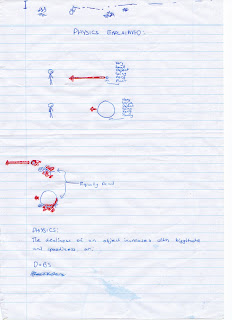rockym93 dot net

Imagine a golf ball...

12 November 200811:59AMscience

So I was in physics today and I decided that instead of doing the revision problems we'd been given, I would write one of my own. It started with a violent stick figure drawing illustrating that a very very small object (like a golf ball) going very very fast does the same amount of damage as a very very large (like a boulder) object going very very slowly.This got me thinking, if the golf ball was moving at the speed of light, how big would the boulder have to be to have the same amount of smearing effect, assuming the boulder is moving at 1m/s.

So:

Golf ball (0.05 kg), 300 000 000 (Speed of light)
1/2 * 0.05 * 300 000 000 ^2 = 2.25x10^15 joules
so 1/2 * x * 1 ^2 = 2.25x10^15 joules x = 5.5x10 ^15 kg or 5 500 000 000 000 tonnes

which is really big.

It sort of loses something in being written down, but I guess what I'm trying to get across is that I've decided to like physics. What other subject lets you smash a golf ball (albeit hypothetical) into things at the speed of light?

< The Joy of Stick Figures Cashiers are human too. >